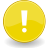# External script memory

 LSL PortalNote! Under Construction More examples need to be added.

There are a few ways in LSL to store data for later use, other than within the script itself. These methods can help to protect data if a script gets inadvertently reset. This page shows some examples of methods that can be used.. Where highly important data is to be held long term, an off-world web-based database is to be preferred.

One way of storing data for later use is llGetObjectDesc and llSetObjectDesc.

<lsl>

default {

```   state_entry()
{
string data = llGetObjectDesc();
if (data)
llSay(0, "I was last touched by " + data);
else
llSay(0, "I have not been touched yet");
}
```
```   touch_start(integer total_number)
{
llResetScript();
}
```

} </lsl>

To create a Boolean constant you can use a method like this:-

<lsl> //These functions should only be used if your script is not going to be using llSetText SetConstant(integer tof){

```   if(tof == TRUE)
llSetPrimitiveParams([ PRIM_TEXT, " ",<1,0,0>, 1.0 ]);
if(tof == FALSE)
llSetPrimitiveParams([ PRIM_TEXT, "",<1,0,0>, 1.0 ]);
}
```

integer GetConstant(){

```  list params = llGetPrimitiveParams([PRIM_TEXT]);
if (llList2String(params,0))
return TRUE;
return FALSE;
```

}

default {

```   state_entry()
{
llSay(0,(string)GetConstant()); //First time should output 0, then once you click and it restarts, it will output 1
}
```
```   touch_start(integer total_number)
{
SetConstant(TRUE);
llResetScript();
}
```

}

</lsl>

Or perhaps more obviously, using the alpha parameter:-

<lsl> //These functions should only be used if your script is not going to be using llSetText SetConstant(integer tof) {

```  llSetPrimitiveParams([ PRIM_TEXT, "", <1,1,1>, tof ]);
```

}

integer GetConstant() {

```  list params = llGetPrimitiveParams([PRIM_TEXT]);
return ( (integer) llList2Float(params, 2) );
```

}

default {

```   state_entry()
{
llSay(0, (string) GetConstant()); // First time will output 1, then on each touch, it toggles between 0 then 1 ...
}

touch_start(integer total_number)
{
integer status = GetConstant();
SetConstant(!status);
llResetScript();
}
```

} </lsl>

Similarly the colour vector could be used to hold more data, but the restrictions on valid colour values will apply (each component in the range 0.0 to 1.0)